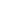科研动态最新资讯

• 天元讲堂（3.11）Spectral Gap for Partially Hyperbolic Systems
• 浏览量：10 发布人： 发布时间：2019-03-11
•   题目：Spectral Gap for Partially Hyperbolic Systems
报告人：陈剑宇（University of Massachusetts, Assistant Professor）
时间：2019年3月11日下午2:00
地点：数学楼二楼报告厅
摘要：It has been a long standing problem on whether generic partially hyperbolic systems have exponential decay of correlations. Typical partially hyperbolic systems include the time-one map of Anosov flows, the skew product over hyperbolic diffeomorphisms, etc.In this talk, we shall focus on the skew products on the torus, which is of the form $F(x, y)=(Tx, y+\tau(x))$. In joint work with Professor Huyi Hu, we show the following dichotomy: (1) Either the transfer operator of $F$ has spectral gap in certain Sobolev space, and thus has exponential decay of correlations; (2) Or the rotation function $\tau$ is an essential coboundary over $T$. The above dichotomy was first established in the case when both $T$ and $\tau$ are $C^\infty$ smooth, and $T$ is uniformly expanding. The key technique we use is the semiclassical analysis of the pseudo-differential operators and Fourier integral operators. Recently, using strong shadowing and stable foliations, we extended this dichotomy for the case when $T$ and $\tau$ are $C^2$ smooth, and $T$ is hyperbolic of solenoid type.